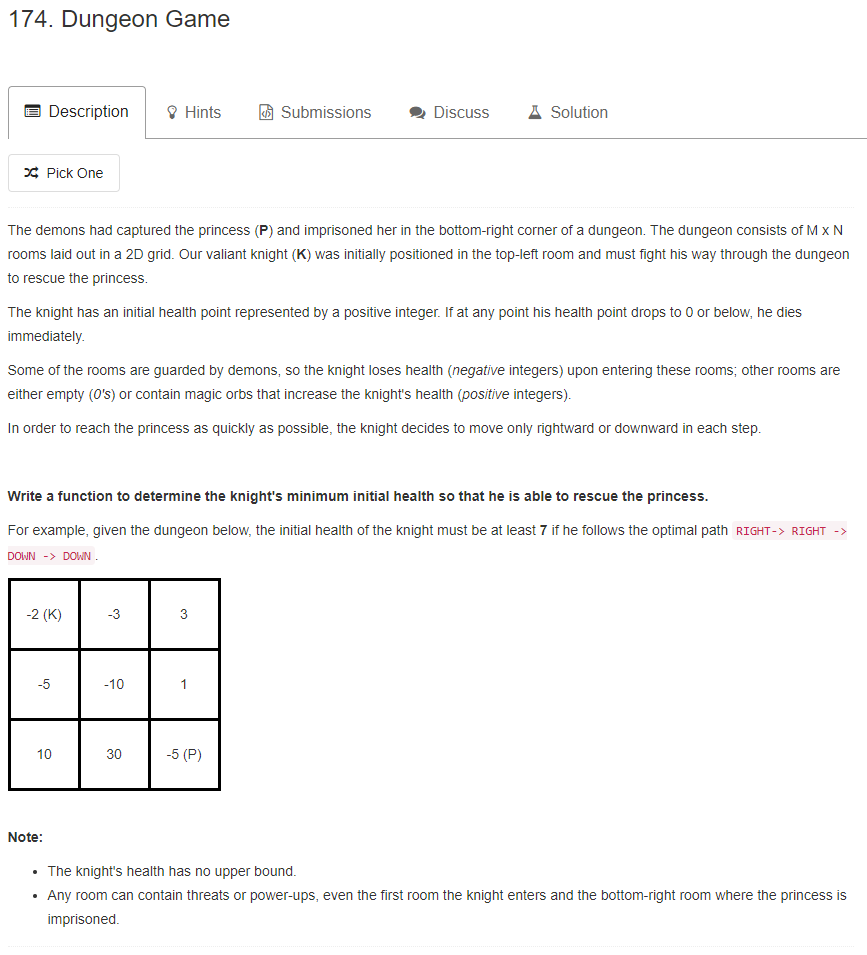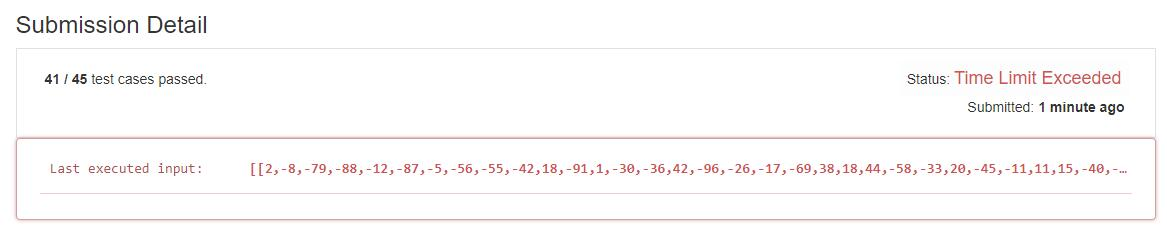# 题目描述（困难难度）# 解法一 回溯法

//全局变量去保存最小值
int minHealth = Integer.MAX_VALUE;

public int calculateMinimumHP(int[][] dungeon) {
//calculateMinimumHPHelper 四个参数
//int x, int y, int health, int addHealth, int[][] dungeon
//x, y 代表要准备到的位置，x 代表是哪一列，y 代表是哪一行
//health 代表当前的生命值
//初始的时候给加 1 点血，addHealth 和 health 都是 1
calculateMinimumHPHelper(0, 0, 1, 1, dungeon);
return minHealth;
}

private void calculateMinimumHPHelper(int x, int y, int health, int addHealth, int[][] dungeon) {
//加上当前位置的奖励或惩罚
health = health + dungeon[y][x];
//此时是否需要加血，加血的话就将 health 加到 1
if (health <= 0) {
}

//是否到了终点
if (x == dungeon.length - 1 && y == dungeon.length - 1) {
return;
}

//是否加过血
if (health <= 0) {
//加过血的话，health 就变为 1
if (x < dungeon.length - 1) {
calculateMinimumHPHelper(x + 1, y, 1, addHealth, dungeon);
}
if (y < dungeon.length - 1) {
calculateMinimumHPHelper(x, y + 1, 1, addHealth, dungeon);
}
} else {
//没加过血的话，health 就是当前的 health
if (x < dungeon.length - 1) {
calculateMinimumHPHelper(x + 1, y, health, addHealth, dungeon);
}
if (y < dungeon.length - 1) {
calculateMinimumHPHelper(x, y + 1, health, addHealth, dungeon);
}
}

}if (addHealth > minHealth) {
return;
}


0 1 2
3 4 5
6 7 8



dp[i][j] 存储从起点 (0, 0) 到达 (i, j) 时候所需要的最小初始生命值。

* * 8 *
* 7 ! ?
? ? ? ?


# 解法二 递归

  ↓
→ *


* → x
↓
y


public int calculateMinimumHP(int[][] dungeon) {
return calculateMinimumHPHelper(0, 0, dungeon);
}

private int calculateMinimumHPHelper(int i, int j, int[][] dungeon) {
//是否到达终点
if (i == dungeon.length - 1 && j == dungeon.length - 1) {
if (dungeon[i][j] > 0) {
return 1;
} else {
return -dungeon[i][j] + 1;
}
}
//右边位置到达终点所需要的最小值，如果已经在右边界，不能往右走了，赋值为最大值
int right = j < dungeon.length - 1 ? calculateMinimumHPHelper(i, j + 1, dungeon) : Integer.MAX_VALUE;
//下边位置到达终点需要的最小值，如果已经在下边界，不能往下走了，赋值为最大值
int down = i < dungeon.length - 1 ? calculateMinimumHPHelper(i + 1, j, dungeon) : Integer.MAX_VALUE;
//当前位置到终点还需要的生命值
int need = right < down ? right - dungeon[i][j] : down - dungeon[i][j];
if (need <= 0) {
return 1;
} else {
return need;
}
}public int calculateMinimumHP(int[][] dungeon) {
return calculateMinimumHPHelper(0, 0, dungeon, new HashMap<String, Integer>());
}

private int calculateMinimumHPHelper(int i, int j, int[][] dungeon, HashMap<String, Integer> map) {
if (i == dungeon.length - 1 && j == dungeon.length - 1) {
if (dungeon[i][j] > 0) {
return 1;
} else {
return -dungeon[i][j] + 1;
}
}
String key = i + "@" + j;
if (map.containsKey(key)) {
return map.get(key);
}
int right = j < dungeon.length - 1 ? calculateMinimumHPHelper(i, j + 1, dungeon, map) : Integer.MAX_VALUE;
int down = i < dungeon.length - 1 ? calculateMinimumHPHelper(i + 1, j, dungeon, map) : Integer.MAX_VALUE;
int need = right < down ? right - dungeon[i][j] : down - dungeon[i][j];
if (need <= 0) {
map.put(key, 1);
return 1;
} else {
map.put(key, need);
return need;
}
}


# 解法三 动态规划

奖惩数组
1   -3   3
0   -2   0
-3  -3   -3

dp 数组

0 0 0 M
0 0 0 M
0 0 4 M
M M M M



public int calculateMinimumHP(int[][] dungeon) {
int row = dungeon.length;
int col = dungeon.length;
int[][] dp = new int[row + 1][col + 1];
//终点所需要的值
dp[row - 1][col - 1] = dungeon[row - 1][col - 1] > 0 ? 1 : -dungeon[row - 1][col - 1] + 1;
//扩充的边界更新为最大值
for (int i = 0; i <= col; i++) {
dp[row][i] = Integer.MAX_VALUE;
}
for (int i = 0; i <= row; i++) {
dp[i][col] = Integer.MAX_VALUE;
}

//逆过来更新
for (int i = row - 1; i >= 0; i--) {
for (int j = col - 1; j >= 0; j--) {
if (i == row - 1 && j == col - 1) {
continue;
}
//选择向右走还是向下走
dp[i][j] = Math.min(dp[i + 1][j], dp[i][j + 1]) - dungeon[i][j];
if (dp[i][j] <= 0) {
dp[i][j] = 1;
}
}
}
return dp;
}


public int calculateMinimumHP(int[][] dungeon) {
int row = dungeon.length;
int col = dungeon.length;
int[] dp = new int[col + 1];

for (int i = 0; i <= col; i++) {
dp[i] = Integer.MAX_VALUE;
}
dp[col - 1] = dungeon[row - 1][col - 1] > 0 ? 1 : -dungeon[row - 1][col - 1] + 1;
for (int i = row - 1; i >= 0; i--) {
for (int j = col - 1; j >= 0; j--) {
if (i == row - 1 && j == col - 1) {
continue;
}
dp[j] = Math.min(dp[j], dp[j + 1]) - dungeon[i][j];
if (dp[j] <= 0) {
dp[j] = 1;
}
}
}
return dp;
}